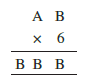# Q7. Find the values of the letters in each of the following and give reasons for the steps involved.The product of 6 & B gives a number whose unit digit is B again.

possible value of B = 0. 2 , 4, 6 or 8

If B = o then our product will be zero. hence this value of B is not possible.

If B = 2, then B x 6 = 12 . Carry for next step = 1.

6A + 1 = BB = 22

implies A = 21/6 = not any integer value hence this case is also not possible.

If B = 6  then B *6 = 36 and 3 will be carry for next step.

6A + 3 = BB = 66

implies A = 63/6 = not an integer value hence this case is also not possible.

If B = 8 then B * 6 = 48 and 4 will be carry for next step.

6A + 4 = BB = 88

implies A = 14 however A is a single digit number hence this case is also not possible.

If B = 4 then B*6 = 24 and 2 will be carry for next step.

6A + 2 = BB = 22

implies A = 7 .

Hence A = 7 and B = 4 is the correct answer.

## Related Chapters

### Preparation Products

##### Knockout NEET May 2021 (One Month)

An exhaustive E-learning program for the complete preparation of NEET..

₹ 14000/- ₹ 6999/-
##### Foundation 2021 Class 10th Maths

Master Maths with "Foundation course for class 10th" -AI Enabled Personalized Coaching -200+ Video lectures -Chapter-wise tests.

₹ 350/- ₹ 112/-
##### Knockout JEE Main April 2021 (One Month)

An exhaustive E-learning program for the complete preparation of JEE Main..

₹ 14000/- ₹ 6999/-
##### Knockout NEET May 2021

An exhaustive E-learning program for the complete preparation of NEET..

₹ 22999/- ₹ 14999/-